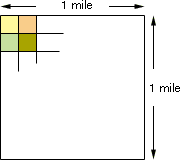Date: Thu, 8 Jul 1999 12:37:39 -0600 (CST)br Sender: Rita
Subject: # of acres in square mile

Question:
# of acres in a square mile

Hi Rita,

 A square mile is a region one mile by one mile, and an acre is a plot of land with an area of 43 560 square feet. One mile is 5280 feet and hence a square mile has area 5280 x 5280 = 27 878 400 square feet. The number of acres in a square mile is thus the number of 43 560 square foot plots it will take to cover this area of 27 878 400 square feet.I hope this helps,
Harley

Go to Math Central